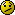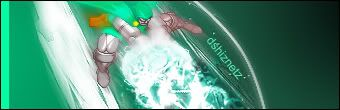# Alex (SF_MX) update (Read 5016 times)

Started by lifido9999, July 31, 2010, 06:46:54 AM

#### lifido9999##### Re: Alex (SF_MX)
#21  August 02, 2010, 04:56:26 AM
••• welcome to SFMX world!
This char was good. But I found some flaws.

-Whats with these sprites?-Theres a typo in the moveslist./\
|
You have to pull off 360 PP to do Hyper Jaguar Revolver.
-The Land spark is misaligned and also when he's lieing down.He's slighty levatating over Mr Bears feet.
-Theres a problem with the Super BG.Maybe MUGEN 1.0 caused it or IDK.

thanks your report. will fix later. before Friday.

#### LaQuak##### Re: Alex (SF_MX)
#22  August 02, 2010, 05:54:24 AM
••Ak you scaled the sprites on top of .cns (I change it to 1/1 on my end it looks much better) and I noticed also that crouching hp twitches to crouching anim if you hold down. Basicaly I would make the attack ChangeState to 0 instead of 11 and problem solved.

Is anybody else having problems doing Ultras? I am pressing double kicks/punches and nothing.#### Luis Alejandro##### Re: Alex (SF_MX)
#23  August 02, 2010, 06:52:20 AM
The only problem i have is that the focus attack doesnt crumble the opponent

great char fido!!!#### Garuda##### Re: Alex (SF_MX)
#24  August 02, 2010, 11:39:50 PM
••• There is a demente in everyone!
To do the ultra, in A groove, the third bar must be full.

#### rednavi -RETIRED##### Re: Alex (SF_MX)
#25  August 03, 2010, 04:13:51 AM
•••Even if his chars behave more like fullgame stuff (in which case i dont see why this is even released as chars to put in your mugen) you must create a command buffering, the other chars must share the same system and every state you go on roundstate must have ctrl 0. if anybody wanna try what im talking about do the next: leave both chars on state 0, pause with p1, check p2 state with debug mode, now u will see that pressing any direction will change p2 state even during the pause.

#### lifido9999##### Re: Alex (SF_MX)
#26  August 04, 2010, 06:36:18 PM
••• welcome to SFMX world!
Too different for me. I just know you are talking about the "pause"

#### lifido9999##### Re: Alex (SF_MX)
#27  August 05, 2010, 05:39:11 PM
••• welcome to SFMX world!
Even if his chars behave more like fullgame stuff (in which case i dont see why this is even released as chars to put in your mugen) you must create a command buffering, the other chars must share the same system and every state you go on roundstate must have ctrl 0. if anybody wanna try what im talking about do the next: leave both chars on state 0, pause with p1, check p2 state with debug mode, now u will see that pressing any direction will change p2 state even during the pause.

I had fixed the channel problem and action problem. thank you!

#### 8way##### Re: Alex (SF_MX)
#28  August 08, 2010, 07:02:35 PM
••oh well, people will never learn that a movelist menu in a char totally breaks other mugen charsCan you please tell me how do you disable it?

#### lifido9999##### Re: Alex (SF_MX)
#29  August 09, 2010, 09:21:45 AM
••• welcome to SFMX world!
oh well, people will never learn that a movelist menu in a char totally breaks other mugen charsCan you please tell me how do you disable it?any botton but S#### 8way##### Re: Alex (SF_MX)
#30  August 09, 2010, 01:37:39 PM
••oh well, people will never learn that a movelist menu in a char totally breaks other mugen charsCan you please tell me how do you disable it?any botton but S??? not sure what you mean, but the problem im having is with some of my characters the the movelist menu will just pop up for no reason and lock up the game, and all i can do is hit esc to end the game. Im looking for the code to delete, but im not sure what im looking for.

#### GT##### Re: Alex (SF_MX) update
#31  August 09, 2010, 02:00:07 PM
You need to open up the CMD and comment this out :

[State -1 ,    pause]
Type = Helper
Trigger1 = NumHelper(10001) = 0
trigger1 =command = "start"
trigger1 = var(25) = 0 || var(26) = 0
Name = "Pause"
ID = 10001
Pos = 0, -600
PosType = Back
StateNo = 10001
KeyCtrl = 1
OwnPal = 1I think. XD
Yeah Titiln, in fact, You Made Him

#### 8way##### Re: Alex (SF_MX) update
#32  August 10, 2010, 03:05:56 AM
••You need to open up the CMD and comment this out :

[State -1 ,    pause]
Type = Helper
Trigger1 = NumHelper(10001) = 0
trigger1 =command = "start"
trigger1 = var(25) = 0 || var(26) = 0
Name = "Pause"
ID = 10001
Pos = 0, -600
PosType = Back
StateNo = 10001
KeyCtrl = 1
OwnPal = 1I think. XD

Thanks, but i dont see anything like that. This is what it looks like:

[Command]
Name = "waza1_light"
Command = ~D, F, x
Time = 9
[Command]
Name = "waza1_middle"
Command = ~D, F, y
Time = 9
[Command]
Name = "waza1_hard"
Command = ~D, F, z
Time = 9

[Command]
Name = "waza2_light"
Command = F, ~D, DF, x
Time = 20
[Command]
Name = "waza2_middle"
Command = F, ~D, DF, y
Time = 20
[Command]
Name = "waza2_hard"
Command = F, ~D, DF, z
Time = 20

[Command]
Name = "waza3_light"
Command = ~D, B, a
[Command]
Name = "waza3_middle"
Command = ~D, B, b
[Command]
Name = "waza3_hard"
Command = ~D, B, c

[Command]
Name = "FF"
Command = F, F
[Command]
Name = "BB"
Command = B, B

[Command]
Name = "a"
Command = a
Time = 1
[Command]
Name = "b"
Command = b
Time = 1
[Command]
Name = "c"
Command = c
Time = 1
[Command]
Name = "x"
Command = x
Time = 1
[Command]
Name = "y"
Command = y
Time = 1
[Command]
Name = "z"
Command = z
Time = 1

[Command]
Name = "start"
Command = s
Time = 1

[Command]
Name = "fwd"
Command = F
Time = 1
[Command]
Name = "back"
Command = B
Time = 1
[Command]
Name = "up"
Command = U
Time = 1
[Command]
Name = "down"
Command = D
Time = 1

[Command]
Name = "holda"
Command = /\$a
Time = 1
[Command]
Name = "holdb"
Command = /\$b
Time = 1
[Command]
Name = "holdc"
Command = /\$c
Time = 1

[Command]
Name = "holdfwd"
Command = /\$F
Time = 1
[Command]
Name = "holdback"
Command = /\$B
Time = 1
[Command]
Name = "holdup"
Command = /\$U
Time = 1
[Command]
Name = "holddown"
Command = /\$D
Time = 1

[Command]
Name = "recovery"
Command = a+b+c+x+y+z+s
Time = 0

[StateDef -1]
[State -1];—³Šªù•—‹r
Type = ChangeState
Trigger1 = (Command="waza3_light"||Command="waza3_middle"||Command="waza3_hard") && (StateType!=A||(Var(13)&&!Var(10))) && (Ctrl||(Time<2&&StateNo=[200,399])||Var(6)>0||(Var(12)=-2&&MoveType=H&&HitShakeOver&&Pos Y<=0)) && Var(30) != 1 && RoundState = 2
Value = IFelse(Command="waza3_light", 1200, IFelse(Command="waza3_middle", 1201, 1202))
[State -1];‹ó’†—³Šªù•—‹r
Type = ChangeState
Trigger1 = (Command="waza3_light"||Command="waza3_middle"||Command="waza3_hard")&&StateType=A&&(Ctrl||Var(6)>0||(Var(12)=-2&&MoveType=H&&HitShakeOver&&Pos Y<=0))&&Pos Y<-55&&Var(10)&&Var(30)!=1&&RoundState=2
Value = IFelse(Command="waza3_light", 1203, IFelse(Command="waza3_middle", 1204, 1205))

[State -1];¸—´Œ
Type = ChangeState
Trigger1 = (Command="waza2_light"||Command="waza2_middle"||Command="waza2_hard") && (StateType!=A||Var(13)) && (Ctrl||(Time<2&&StateNo=[200,399])||Var(6)>0||(Var(12)=-2&&MoveType=H&&HitShakeOver&&Pos Y<=0)) && Var(30) != 1 && RoundState = 2
Value = IFelse(Command="waza2_light", 1100, IFelse(Command="waza2_middle", 1101, 1102))

[State -1];”g“®Œ
Type = ChangeState
Trigger1=(Command="waza1_light"||Command="waza1_middle"||Command="waza1_hard")&&!NumProjID(1)&&(StateType!=A||Var(13))&&(Ctrl||(Time<2&&StateNo=[200,399])||Var(6)>0||(Var(12)=-2&&MoveType=H&&HitShakeOver&&Pos Y<=0))&&Var(30)!=1&&RoundState=2
Value = IFelse(Command="waza1_light", 1000, IFelse(Command="waza1_middle", 1001, 1002))

[State -1];“Š‚°
Type = ChangeState
Trigger1 = StateNo = 20 && (Command = "y" || Command = "z" || Command = "b" || Command = "c" || (Var(10) = 2 && Command = "a")) && P2BodyDist X<(65*Const(Size.XScale)) && Var(30) != 1 && RoundState = 2
Value = IFelse(Command = "y", 504, IFelse(Command = "z", 500, IFelse(Command = "b", 510, 514)))

[State -1];—§‚¿ƒpƒ“ƒ`ŒnUŒ‚
Type = ChangeState
Trigger1 = (Command = "x" || Command = "y" || Command = "z") && StateType = S && (Ctrl||(Var(12)=-2&&MoveType=H&&HitShakeOver)) && Var(30) != 1 && RoundState = 2
Value = IFelse(Command="x", 200+(P2BodyDist X<(35*Const(Size.XScale))), IFelse(Command="y", 210+(P2BodyDist X<(42*Const(Size.XScale))), 220+(P2BodyDist X<(46*Const(Size.XScale)))))
[State -1];—§‚¿ƒLƒbƒNŒnUŒ‚
Type = ChangeState
Trigger1 = (Command = "a" || Command = "b" || Command = "c") && StateType = S && (Ctrl||(Var(12)=-2&&MoveType=H&&HitShakeOver)) && Var(30) != 1 && RoundState = 2
Value = IFelse(P2BodyDist X>(29*Const(Size.XScale)), IFelse(Command = "a", 230, IFelse(Command = "b", 240, IFelse(P2BodyDist X<(45*Const(Size.XScale)), 251, 250))), IFelse(Command = "a", 231, IFelse(Command = "b", 241, 251)))
[State -1];‚µ‚á‚ª‚ÝUŒ‚
Type = ChangeState
Trigger1 = (Command = "x" || Command = "y" || Command = "z" || Command = "a" || Command = "b" || Command = "c") && StateType = C && (Ctrl||(Var(12)=-2&&MoveType=H&&HitShakeOver)) && Var(30) != 1 && RoundState = 2
Value = IFelse(Command = "x", 300, IFelse(Command = "y", 310, IFelse(Command = "z", 320, IFelse(Command = "a", 330, IFelse(Command = "b", 340, 350)))))
[State -1];‹ó’†ƒpƒ“ƒ`
Type = ChangeState
Trigger1 = (Command = "x" || Command = "y" || Command = "z") && StateType = A && (Ctrl||(Var(12)=-2&&MoveType=H&&HitShakeOver&&Pos Y<=0)) && Var(30) != 1 && RoundState = 2
Value = IFelse(Command = "x", 400, IFelse(Command = "y", 410, 420))
[State -1];‹ó’†ƒLƒbƒN
Type = ChangeState
Trigger1 = (Command = "a" || Command = "b" || Command = "c") && StateType = A && (Ctrl||(Var(12)=-2&&MoveType=H&&HitShakeOver&&Pos Y<=0)) && Var(30) != 1 && RoundState = 2
Value = IFelse(Vel X = 0, IFelse(Command = "a", 430, IFelse(Command = "b", 440, 450)), IFelse(Command = "a", 431, IFelse(Command = "b", 441, 451)))
[State -1];“¯Žž‰Ÿ‚µƒXƒyƒVƒƒƒ‹
Type = ChangeState
Trigger1 = Command = "a" && AnimElem = 2,>1 && (Command="x"||Command="y"||Command="z"||Command="holdup") && ((StateNo=231&&Command="holddown") || (Command!="holddown"&&StateNo=330)) && RoundState = 2
Value=IFelse(Command="holdup",40,IFelse(Command="holddown",IFelse(Command="x",300,IFelse(Command="y",310,320)),IFelse(P2Dist X<60*Const(Size.XScale),IFelse(Command="x",201,IFelse(Command="y",211,221)),IFelse(Command="x",200,IFelse(Command="y",210,220)))))
[State -1];ŽãUŒ‚˜A‘Åˆ—
Type = ChangeState
Trigger1 = (Command="x"&&((StateNo=[200,201])||StateNo=300)&&AnimElem=2,>1) || ((Command = "holddown" || P2Dist X < 60*Const(Size.XScale)) && Command = "a" && ((StateNo = 231 && AnimElem = 2,>1) || (StateNo = 330 && AnimElem = 2,>1)))&&RoundState=2
Value = IFelse(Command="x", IFelse(Command="holddown", 300, IFelse(P2Dist X>59*Const(Size.XScale),200,201)), IFelse(Command="holddown", 330, IFelse(StateNo=330||P2Dist X<60*Const(Size.XScale), 231, 230)))